Next: Bibliography

Project:
Hybrid Coupling

 Researcher(s) : Prof. Dr. R. Hiptmair : Prof. Dr. Ana Alonso-Rodriguez, Universita Degli Studi Di Trento, Ital, : Prof. Dr. Alberto Valli, Universita Degli Studi Di Trento, Italy Funding : no external funding Duration : ongoing project

Description. The general principle underlying hybrid coupling can best be explained in the case the scalar model problem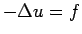in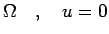on(1)

for some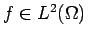. This way to write the boundary value problem is called the primal formulation. The corresponding dual formulation relies on the first order system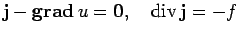inon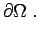(2)

This is sometimes referred to as the mixed formulation of (1). Primal and dual formulations give rise to different variational problems. It is the gist of the hybrid coupling approach to employ these two variational formulations on different subdomains of a partition of the computational domain. An important restriction is that the same formulation must not be used on adjacent sub-domains.

Let us assume that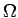is partitioned into two connected Lipschitz subdomains(primal'') and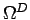(dual'') such that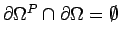. Let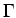be their common interface, with the unit normal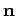onpointing into. Onwe resort to the primal variational formulation obtained from integration by parts applied to (1): seek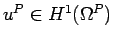such that(3)

Onthe mixed variational formulation will be used, which emerges from casting the first equation of (2) into weak form and retaining the second strongly: seek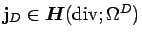,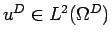such that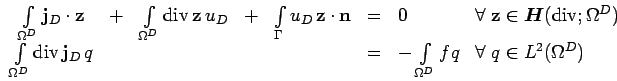(4)

(remember thatis the unit inward normal vector on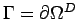).

Both problems can be linked by the transmission conditions on: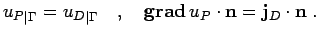(5)

These can be used to express the interface terms in both (3) and (4) through quantities from the other subdomain. Subsequently merging the variational formulations we arrive at the final coupled problem: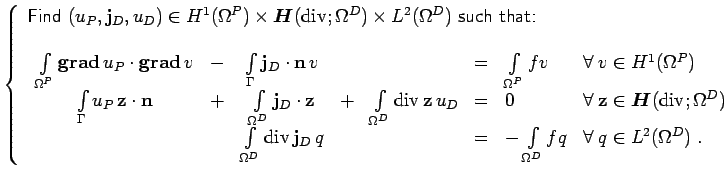(6)

Let us denote by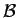the bilinear form oncorresponding to the left-upper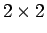block of (6). The crucial observation is thatitself features a block-skew-symmetric structure, which involves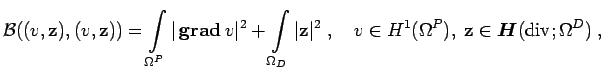(7)

because the interface contributions cancel. This makes it possible to show thatsatisfies an inf-sup condition on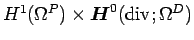. Beware, that this is not straightforward from (7), because the one-dimensional topological subspace of constants onrequires a special treatment. Then the theory of saddle-point problems confirms existence and uniqueness of solutions of (7). Moreover, Galerkin approximations by the standard conforming finite element spaces for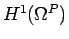,and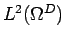will enjoy optimality [WW98].

It is important to note that the coupling of the two subdomains in (7) is purely variational, because none of the transmission conditions (5) shows up in the definition of the spaces. This makes (7) attractive, if unrelated (non-matching'') finite element meshes are to be used onand.

Hybrid coupling for eddy current problems. The eddy current equations in frequency domain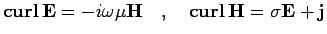give rise to another example of a second order boudary value problem. The hybrid coupling idea outlined above can also be applied to this system, though the theoretical analysis becomes more involved [AHV04,ARHV04]. The reason is that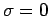is encountered in non-conducting regions.

Hybrid domain decomposition on non-matching grids. We want to resort to the hybrid coupling idea to deal with non-matching grids arising from a partitioning ofinto subdomains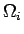, across whose boundaries the finite element meshes need not match see Fig. 1.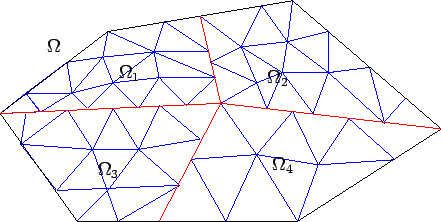The idea is to introduce narrow stripes of width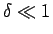along the interfaces, see Fig. 2. In these stripes the problem is cast into primal form, whereas in the remainder of the domain we use the mixed form. Letting width of the stripes tend to zero, we hope to obtain a mixed formulation on the non-matching meshes.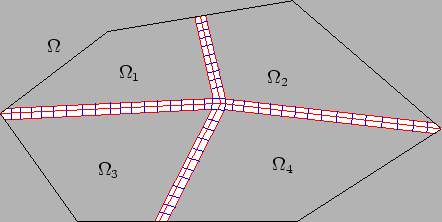Next: Bibliography
Prof. Ralf Hiptmair 2004-11-11